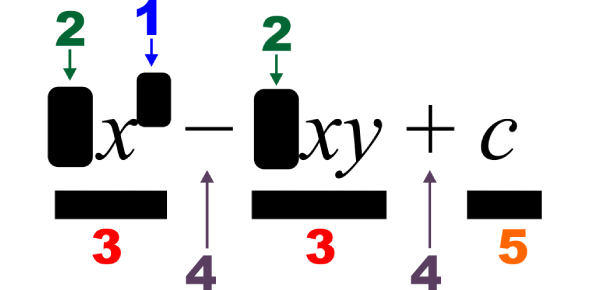# Literal Equations Quiz

10 Questions | Attempts: 7715
ShareSettingsHow good are you with literal equations? How well can you score in this literal equations quiz? A literal equation is meant by an equation that consists primarily of letters. Formulas are a very big example of literal equations. Each variable in the equation "literally" shows an important part of the whole relationship expressed by the equation. Give this quiz a try, and you will further hone your math skills. Good luck!Back to top